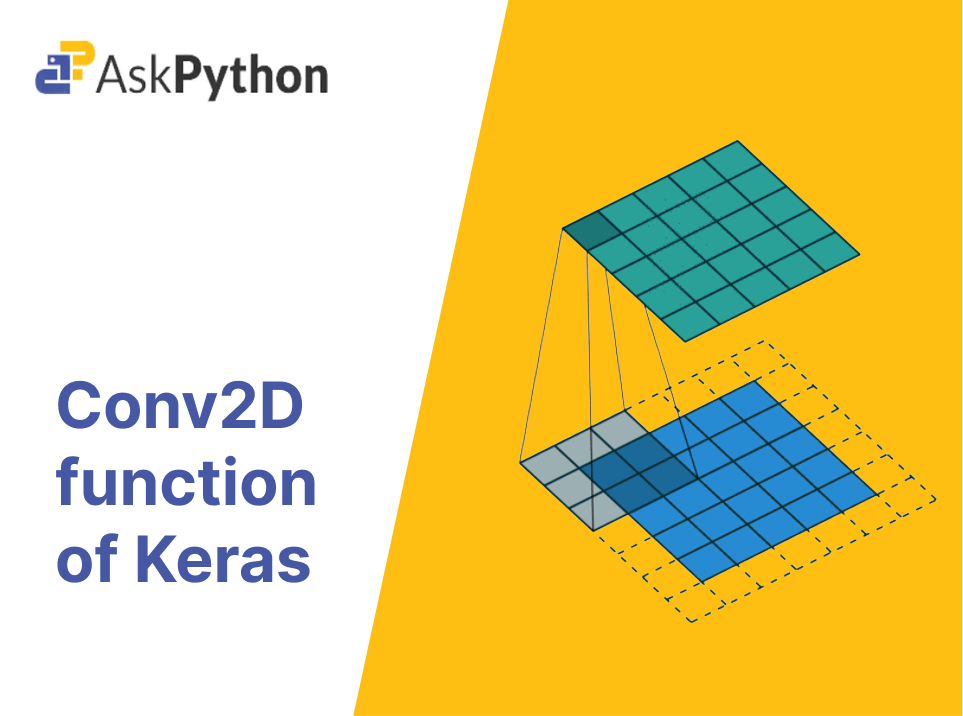# Conv2D function of Keras and its use in CNNsConv2D is a Keras function that is widely used in building CNNs for image processing tasks. In this article, we will discuss Conv2D in detail, including its working principle, code implementation examples, and practical use cases.

Find more on use of Keras in Deep Learning here

### What is Convolution operation?Convolution Operation using a 3×3 filter on a 5×5 image having a stride of 1

The convolution operation is a fundamental building block of CNNs. The idea behind convolution is to apply a set of filters to an input image, with each filter representing a specific feature of the image. The output of the convolution operation is a set of feature maps, which can be further processed by additional layers of the CNN. The convolution operation involves two main components: the kernel and the stride.

The kernel is a small matrix of weights that is applied to the input image. The size of the kernel is typically smaller than the input image, and its values are learned during the training process. The kernel slides over the input image, and at each position, it computes the dot product between its values and the corresponding values of the input image.

### What is Conv2D?

Conv2D is a function provided by the Keras library that performs a 2D convolution operation on input images. It is a building block for building convolutional neural networks. Conv2D is designed to learn features or patterns in an input image by applying a set of learnable filters on the input image. The filters are learned through the training process, which allows the model to learn the most relevant features from the input image for a given task.

The Conv2D function takes several parameters, including the number of filters, the size of the filters, the activation function, and the padding mode. Let’s take a closer look at each of these parameters.

### Parameters of Conv2D

• Number of filters: The number of filters is the number of learnable feature maps that the Conv2D function applies to the input image. Each filter detects a specific feature or pattern in the input image. The more filters, the more features the model can learn from the input image.
• Size of filters: The size of filters is the dimension of the learnable filters. For example, a 3×3 filter means that the filter is a 3×3 matrix, and it slides across the input image to detect patterns.
• Activation function: The activation function is applied to the output of the Conv2D operation. It adds non-linearity to the model, allowing the model to learn complex features and patterns.
• Padding mode: The padding mode is used to control the output size of the Conv2D operation. It is important to maintain the size of the output image when building a CNN. There are two padding modes: ‘valid’ and ‘same.’ ‘Valid’ means no padding is applied, and the output image size is reduced. ‘Same’ means padding is applied to the input image, and the output size is the same as the input size.

### Implementing Conv2D on an image

To better understand Conv2D, let’s implement it on a sample image. We will be using the CIFAR-10 dataset, which consists of 60,000 32×32 color images in 10 classes, with 6,000 images per class.

First, we need to import the necessary libraries and load the dataset.

```import tensorflow as tf
from tensorflow import keras

(x_train, y_train), (x_test, y_test) = keras.datasets.cifar10.load_data()
```

Now, let’s normalize the input data by dividing each pixel by 255.

```x_train = x_train.astype('float32') / 255.0
x_test = x_test.astype('float32') / 255.0

```

Next, we need to define our model using the Keras Sequential API

```model = keras.Sequential([
keras.layers.MaxPooling2D((2,2)),
keras.layers.MaxPooling2D((2,2)),
keras.layers.MaxPooling2D((2,2)),
keras.layers.Flatten(),
keras.layers.Dense(128, activation='relu'),
keras.layers.Dense(10)])

```

Our model consists of three convolutional layers, each followed by a max pooling layer. The first convolutional layer has 32 filters with a size of 3×3 and a ‘same’ padding mode. The second convolutional layer has 64 filters with a size of 3×3 and a ‘same’ padding mode. The third convolutional layer has 128 filters with a size of 3×3 and a ‘same’ padding mode.

After the convolutional layers, we have a flatten layer, which flattens the output of the previous layer into a 1D vector. This output is then passed through two dense layers, with the first one having 128 units and a ReLU activation function, and the last one having 10 units without any activation function. The final layer outputs the probabilities of the 10 classes.

Finally, we need to compile the model by specifying the loss function, optimizer, and metrics.

```model.compile(optimizer='adam',
loss=tf.keras.losses.SparseCategoricalCrossentropy(from_logits=True),
metrics=['accuracy'])
```

Now, we can train the model using the fit method.

```history = model.fit(x_train, y_train, epochs=10,
validation_data=(x_test, y_test))
```

After training the model, we can evaluate it on the test set.

```test_loss, test_acc = model.evaluate(x_test, y_test, verbose=2)
print('Test accuracy:', test_acc)
```

### Conclusion

In this article, we looked at the Conv2D layer or Keras, its parameters and implementation in a CNN model. You can find other such articles at AskPython

References : Official documentation of Conv2D Keras